# Balbharati solutions for Mathematics 2 Geometry 10th Standard SSC Maharashtra State Board chapter 2 - Pythagoras Theorem [Latest edition]

#### Chapters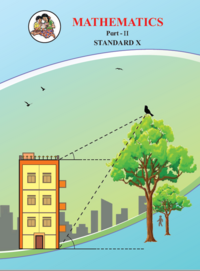## Chapter 2: Pythagoras Theorem

Practice Set 2.1Practice Set 2.2Problem Set 2
Practice Set 2.1 [Pages 38 - 39]

### Balbharati solutions for Mathematics 2 Geometry 10th Standard SSC Maharashtra State Board Chapter 2 Pythagoras Theorem Practice Set 2.1 [Pages 38 - 39]

Practice Set 2.1 | Q 1.1 | Page 38

Identify, with reason, if the following is a Pythagorean triplet.
(3, 5, 4)

Practice Set 2.1 | Q 1.2 | Page 38

Identify, with reason, if the following is a Pythagorean triplet.
(4, 9, 12)

Practice Set 2.1 | Q 1.3 | Page 38

Identify, with reason, if the following is a Pythagorean triplet.
(5, 12, 13)

Practice Set 2.1 | Q 1.4 | Page 38

Identify, with reason, if the following is a Pythagorean triplet.
(24, 70, 74)

Practice Set 2.1 | Q 1.5 | Page 38

Identify, with reason, if the following is a Pythagorean triplet.
(10, 24, 27)

Practice Set 2.1 | Q 1.6 | Page 38

Identify, with reason, if the following is a Pythagorean triplet.
(11, 60, 61)

Practice Set 2.1 | Q 2 | Page 38

In the given figure, ∠MNP = 90°, seg NQ ⊥seg MP, MQ = 9, QP = 4, find NQ.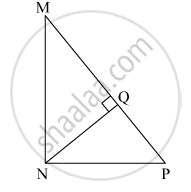Practice Set 2.1 | Q 3 | Page 38

In the given figure, ∠QPR = 90°, seg PM ⊥ seg QR and Q–M–R, PM = 10, QM = 8, find QR.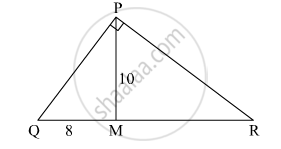Practice Set 2.1 | Q 4 | Page 39

In the given figure. Find RP and PS using the information given in ∆PSR.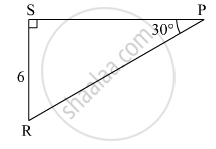Practice Set 2.1 | Q 5 | Page 39

For finding AB and BC with the help of information given in the figure, complete following activity.

AB = BC ..........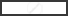$\therefore \angle BAC =$$\therefore AB = BC =$$\times AC$

$=$$\times \sqrt{8}$

$=$$\times 2\sqrt{2}$

=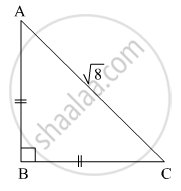Practice Set 2.1 | Q 6 | Page 39

Find the side and perimeter of a square whose diagonal is 10 cm ?

Practice Set 2.1 | Q 7 | Page 39

In the given figure, ∠DFE = 90°, FG ⊥ ED, If GD = 8, FG = 12, find (1) EG (2) FD and (3) EF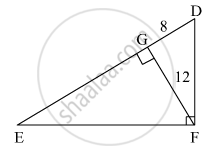Practice Set 2.1 | Q 8 | Page 39

Find the diagonal of a rectangle whose length is 35 cm and breadth is 12 cm.

Practice Set 2.1 | Q 9 | Page 39

In the given figure, M is the midpoint of QR. ∠PRQ = 90°. Prove that, PQ= 4PM– 3PR2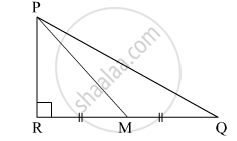Practice Set 2.1 | Q 10 | Page 39

Walls of two buildings on either side of a street are parellel to each other. A ladder 5.8 m long is placed on the street such that its top just reaches the window of a building at the height of 4 m. On turning the ladder over to the other side of the street , its top touches the window of the other building at a height 4.2 m. Find the width of the street.

Practice Set 2.2 [Page 43]

### Balbharati solutions for Mathematics 2 Geometry 10th Standard SSC Maharashtra State Board Chapter 2 Pythagoras Theorem Practice Set 2.2 [Page 43]

Practice Set 2.2 | Q 1 | Page 43

In ∆PQR, point S is the midpoint of side QR. If PQ = 11, PR = 17, PS = 13, find QR.

Practice Set 2.2 | Q 2 | Page 43

In ∆ABC, AB = 10, AC = 7, BC = 9 then find the length of the median drawn from point C to side AB

Practice Set 2.2 | Q 3 | Page 43

In the given figure seg PS is the median of ∆PQR and PT ⊥ QR. Prove that,

${PR}^2 = {PS}^2 + QR \times ST + \left( \frac{QR}{2} \right)^2$

${PQ}^2 = {PS}^2 - QR \times ST + \left( \frac{QR}{2} \right)^2$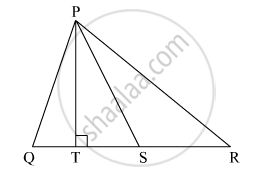Practice Set 2.2 | Q 4 | Page 43

In ∆ABC, point M is the midpoint of side BC.
If, AB+ AC= 290 cm2, AM = 8 cm, find BC.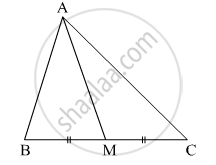Practice Set 2.2 | Q 5 | Page 43

In the given figure, point T is in the interior of rectangle PQRS, Prove that, TS+ TQ= TP+ TR(As shown in the figure, draw seg AB || side SR and A-T-B)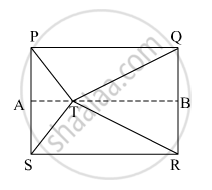Problem Set 2 [Pages 43 - 46]

### Balbharati solutions for Mathematics 2 Geometry 10th Standard SSC Maharashtra State Board Chapter 2 Pythagoras Theorem Problem Set 2 [Pages 43 - 46]

Problem Set 2 | Q 1.1 | Page 43

Some question and their alternative answer are given. Select the correct alternative.

Out of the following which is the Pythagorean triplet?

• (1, 5, 10)

• (3, 4, 5)

• (2, 2, 2)

• (5, 5, 2)

Problem Set 2 | Q 1.2 | Page 43

Some question and their alternative answer are given.

In a right-angled triangle, if sum of the squares of the sides making right angle is 169 then what is the length of the hypotenuse?

• 15

• 13

• 12

Problem Set 2 | Q 1.3 | Page 43

Some question and their alternative answer are given. Select the correct alternative.

Out of the dates given below which date constitutes a Pythagorean triplet ?

• 15/08/17

• 16/08/16

• 3/5/17

• 4/9/15

Problem Set 2 | Q 1.4 | Page 43

Some question and their alternative answer are given. Select the correct alternative.

If a, b, c are sides of a triangle and a+ b= c2, name the type of triangle.

• Obtuse angled triangle

• Acute angled triangle

• Right angled triangle

• Equilateral triangle

Problem Set 2 | Q 1.5 | Page 43

Some question and their alternative answer are given. Select the correct alternative.

Find perimeter of a square if its diagonal is $10\sqrt{2}$

• 10 cm

•  $40\sqrt{2}$cm

• 20 cm

• 40 cm

Problem Set 2 | Q 1.6 | Page 43

Some question and their alternative answer are given. Select the correct alternative.

Altitude on the hypotenuse of a right angled triangle divides it in two parts of lengths 4 cm and 9 cm. Find the length of the altitude.

• 9 cm

• 4 cm

• 6 cm

• $2\sqrt{6}$ cm

Problem Set 2 | Q 1.7 | Page 43

Some question and their alternative answer are given. Select the correct alternative.

Height and base of a right angled triangle are 24 cm and 18 cm find the length of its hypotenuse

• 24 cm

• 30 cm

• 15 cm

• 18 cm

Problem Set 2 | Q 1.8 | Page 43

Some question and their alternative answer are given. Select the correct alternative.

In ∆ABC, AB = $6\sqrt{3}$ cm, AC = 12 cm, BC = 6 cm. Find measure of ∠A.

• 30°

• 60°

• 90°

• 45°

Problem Set 2 | Q 2.1 | Page 44

Solve the following example.

Find the height of an equilateral triangle having side 2a.

Problem Set 2 | Q 2.2 | Page 44

Do sides 7 cm, 24 cm, 25 cm form a right angled triangle ? Give reason

Problem Set 2 | Q 2.3 | Page 44

Find the length a diagonal of a rectangle having sides 11 cm and 60 cm.

Problem Set 2 | Q 2.4 | Page 44

Find the length of the hypotenuse of a right angled triangle if remaining sides are 9 cm and 12 cm.

Problem Set 2 | Q 2.5 | Page 44

A side of an isosceles right angled triangle is x. Find its hypotenuse.

Problem Set 2 | Q 2.6 | Page 44

In ∆PQR; PQ =$\sqrt{8}$, QR =$\sqrt{5}$, PR = $\sqrt{3}$. Is ∆PQR a right angled triangle ? If yes, which angle is of 90°?

Problem Set 2 | Q 3 | Page 44

In ∆RST, ∠S = 90°, ∠T = 30°, RT = 12 cm then find RS and ST.

Problem Set 2 | Q 4 | Page 44

Find the diagonal of a rectangle whose length is 16 cm and area is 192 sq.cm ?

Problem Set 2 | Q 5 | Page 44

Find the length of the side and perimeter of an equilateral triangle whose height is $\sqrt{3}$ cm.

Problem Set 2 | Q 6 | Page 44

In ∆ABC seg AP is a median. If BC = 18, AB2 + AC2 = 260 Find AP.

Problem Set 2 | Q 7 | Page 45

∆ABC is an equilateral triangle. Point P is on base BC such that PC = $\frac{1}{3}$ BC, if AB = 6 cm find AP.

Problem Set 2 | Q 8 | Page 45

From the information given in the figure, prove that PM = PN =  $\sqrt{3}$  × a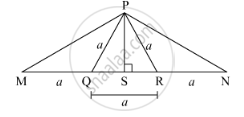Problem Set 2 | Q 9 | Page 45

Prove that the sum of the squares of the diagonals of a parallelogram is equal to the sum of the squares of its sides.

Problem Set 2 | Q 10 | Page 45

Pranali and Prasad started walking to the East and to the North respectively, from the same point and at the same speed. After 2 hours distance between them was $15\sqrt{2}$

km. Find their speed per hour.

Problem Set 2 | Q 11 | Page 45

In ∆ABC, ∠BAC = 90°, seg BL and seg CM are medians of ∆ABC. Then prove that:
4(BL+ CM2) = 5 BC2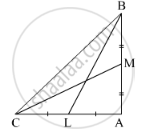Problem Set 2 | Q 12 | Page 45

Sum of the squares of adjacent sides of a parallelogram is 130 sq.cm and length of one of its diagonals is 14 cm. Find the length of the other diagonal.

Problem Set 2 | Q 13 | Page 45

In ∆ABC, seg AD ⊥ seg BC DB = 3CD. Prove that :
2AB= 2AC+ BC2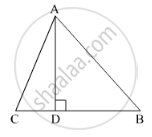Problem Set 2 | Q 14 | Page 44

In an isosceles triangle, length of the congruent sides is 13 cm and its base is 10 cm. Find the distance between the vertex opposite the base and the centroid.

Problem Set 2 | Q 15 | Page 46

In a trapezium ABCD, seg AB || seg DC seg BD ⊥ seg AD, seg AC ⊥ seg BC, If AD = 15, BC = 15 and AB = 25. Find A(▢ABCD)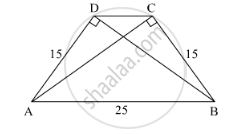Problem Set 2 | Q 16 | Page 44

In the given figure, ∆PQR is an equilateral triangle. Point S is on seg QR such thatn QS =n$\frac{1}{3}$ QR.

Prove that : 9 PS= 7 PQ2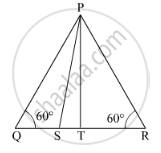Problem Set 2 | Q 17 | Page 44

Seg PM is a median of ∆PQR. If PQ = 40, PR = 42 and PM = 29, find QR.

Problem Set 2 | Q 18 | Page 46

Seg AM is a median of ∆ABC. If AB = 22, AC = 34, BC = 24, find AM

## Chapter 2: Pythagoras Theorem

Practice Set 2.1Practice Set 2.2Problem Set 2## Balbharati solutions for Mathematics 2 Geometry 10th Standard SSC Maharashtra State Board chapter 2 - Pythagoras Theorem

Balbharati solutions for Mathematics 2 Geometry 10th Standard SSC Maharashtra State Board chapter 2 (Pythagoras Theorem) include all questions with solution and detail explanation. This will clear students doubts about any question and improve application skills while preparing for board exams. The detailed, step-by-step solutions will help you understand the concepts better and clear your confusions, if any. Shaalaa.com has the Maharashtra State Board Mathematics 2 Geometry 10th Standard SSC Maharashtra State Board solutions in a manner that help students grasp basic concepts better and faster.

Further, we at Shaalaa.com provide such solutions so that students can prepare for written exams. Balbharati textbook solutions can be a core help for self-study and acts as a perfect self-help guidance for students.

Concepts covered in Mathematics 2 Geometry 10th Standard SSC Maharashtra State Board chapter 2 Pythagoras Theorem are Apollonius Theorem, Application of Pythagoras Theorem in Acute Angle and Obtuse Angle, Converse of Pythagoras Theorem, Theorem of geometric mean, Similarity in Right Angled Triangles, 30 - 60 - 90 and 45 - 45 - 90 Theorem, Right-angled Triangles and Pythagoras Property.

Using Balbharati 10th Standard Board Exam solutions Pythagoras Theorem exercise by students are an easy way to prepare for the exams, as they involve solutions arranged chapter-wise also page wise. The questions involved in Balbharati Solutions are important questions that can be asked in the final exam. Maximum students of Maharashtra State Board 10th Standard Board Exam prefer Balbharati Textbook Solutions to score more in exam.

Get the free view of chapter 2 Pythagoras Theorem 10th Standard Board Exam extra questions for Mathematics 2 Geometry 10th Standard SSC Maharashtra State Board and can use Shaalaa.com to keep it handy for your exam preparation